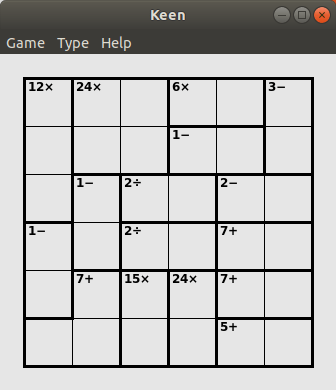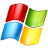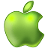# Keen – Complete the Latin Square – Puzzle Game

### KeenYou have a square grid; each square may contain a digit from 1 to the size of the grid. The grid is divided into blocks of varying shape and size, with arithmetic clues written in them. Your aim is to fully populate the grid with digits such that:

• Each row contains only one occurrence of each digit
• Each column contains only one occurrence of each digit
• The digits in each block can be combined to form the number stated in the clue, using the arithmetic operation given in the clue. That is:
• An addition clue means that the sum of the digits in the block must be the given number. For example, ‘15+’ means the contents of the block adds up to fifteen
• A multiplication clue (e.g. ‘60×’), similarly, means that the product of the digits in the block must be the given number
• A subtraction clue will always be written in a block of size two, and it means that one of the digits in the block is greater than the other by the given amount. For example, ‘2−’ means that one of the digits in the block is 2 more than the other, or equivalently that one digit minus the other one is 2. The two digits could be either way round, though
• A division clue (e.g. ‘3÷’), similarly, is always in a block of size two and means that one digit divided by the other is equal to the given amount

Game modes:

• 4×4 – Easy
• 5×5 – Easy
• 6×6 – Easy
• 6×6 – Normal
• 6×6 – Normal
• 6×6 – Hard
• 6×6 – Extreme
• 6×6 – Unreasonable
• 9×9 – Normal
• Custom mode

This puzzle was invented by Tetsuya Miyamoto in 2004. It is known as KenKen. The name derives from the Japanese word for cleverness. The New York Times publishes 6 new KenKen puzzles daily.

Below is a short tutorial showing you how to download and compile the latest source code for this game, and the other 38 games in the puzzle collection. The tutorial takes place in Linux, using the Ubuntu 17.10 distribution, but the procedure will be the same, or very similar, for other Linux distributions. If you find the tutorial helpful, please give the video a like and subscribe to our YouTube channel.

 OS Supported NotesBesides the main 3 operating system, the puzzle collection has also been ported by contributors to Palm, Android, Symbian 60, iPhone, iPad, and the Windows Store. Homepage: www.chiark.greenend.org.uk/~sgtatham/puzzles/ Developer: Simon Tatham, Richard Boulton, James Harvey, Mike Pinna, Jonas Kölker, Dariusz Olszewski, Michael Schierl, Lambros Lambrou, Bernd Schmidt, Steffen Bauer, Lennard Sprong, and Rogier Goossens License: MIT Written in: C, Java, JavaScriptBack to the Puzzle Collection